Get instant live expert help with Excel or Google Sheets“My Excelchat expert helped me in less than 20 minutes, saving me what would have been 5 hours of work!”

#### Post your problem and you’ll get Expert help in seconds.

Your message must be at least 40 characters
Our professional Expert are available now. Your privacy is guaranteed.

# How to Calculate Elapsed Time in Excel

Calculating the elapsed time or time difference is easily done in Excel.  We simply subtract the before and after time, ensuring the correct or preferred time format.  This tutorial will step through the simple steps to calculate elapsed time in Excel.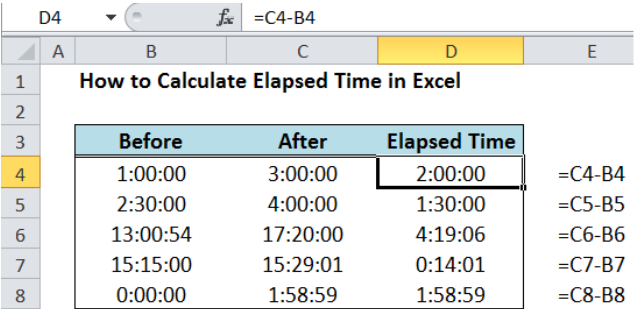Figure 1. Calculating elapsed time in Excel

## Setting up the Data

• Create a table with Before, After and Elapsed Time headers.  Enter the examples as shown in the table below.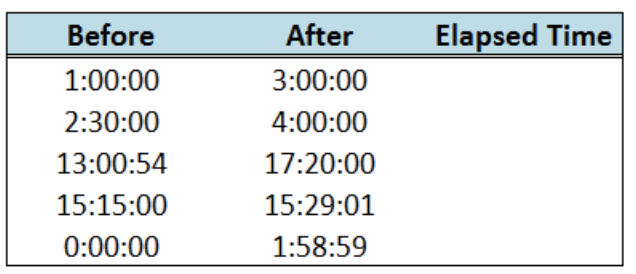Figure 2.  Sample Data for Calculating Elapsed Time in Excel

• Select the data range for the table.  In this case, select cells B4:D8.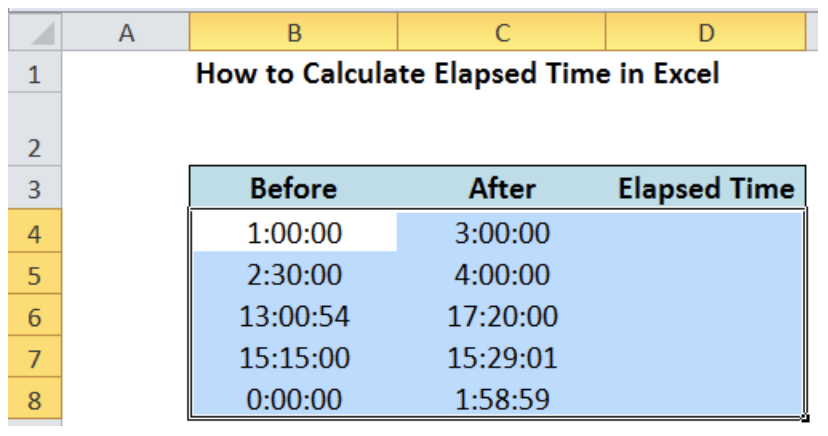Figure 3.  Selection of the data range to set the time format

• Press the keys Ctrl + 1 to open the Format Cells dialog box
• Select the time format “h:mm:ss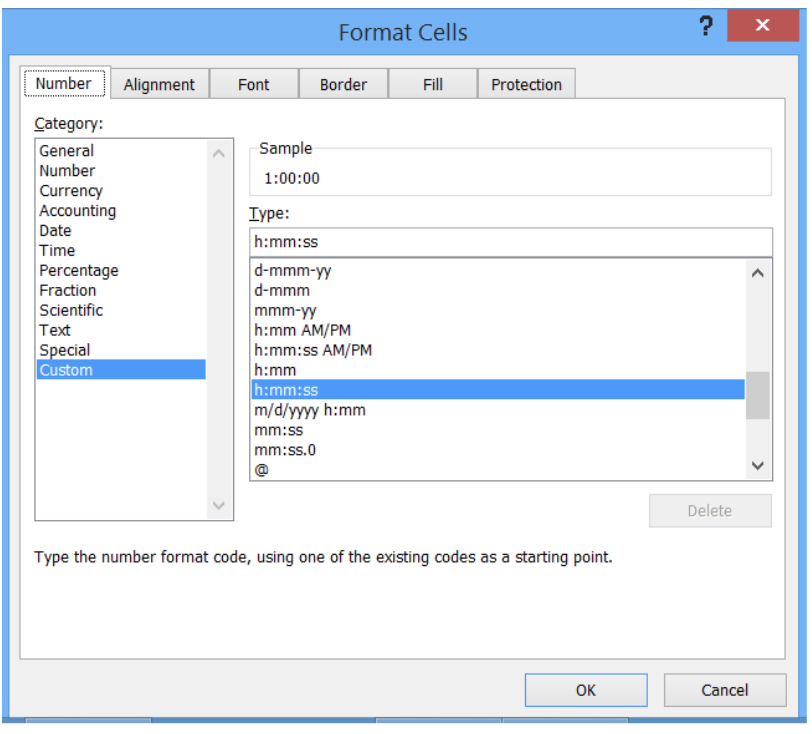Figure 4.  Selection of the preferred time format

## Calculate Elapsed Time

### Formula :

`Elapsed Time = “After” - “Before”`

It’s that simple.  The trick is in ensuring that the time format used is the same to avoid confusion.

In cell D4, enter the formula:

`=C4-B4`

`=“3:00:00”  - “1:00:00”`

The result is 2:00:00 or 2 hours.

Let us do the same for the data in rows 5 to 8.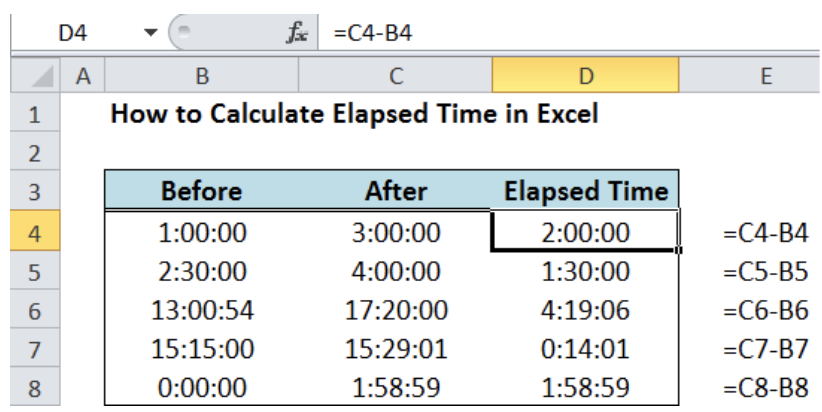Figure 5.  Output: Calculating elapsed time in Excel

### Notes:

1. We can change the format of the elapsed time according to our preference.  Let’s take the last example and express the elapsed time in three different time formats: h:mm, h:mm:ss, mm:ss.

Note that the value shown for the elapsed time differ depending on the format used.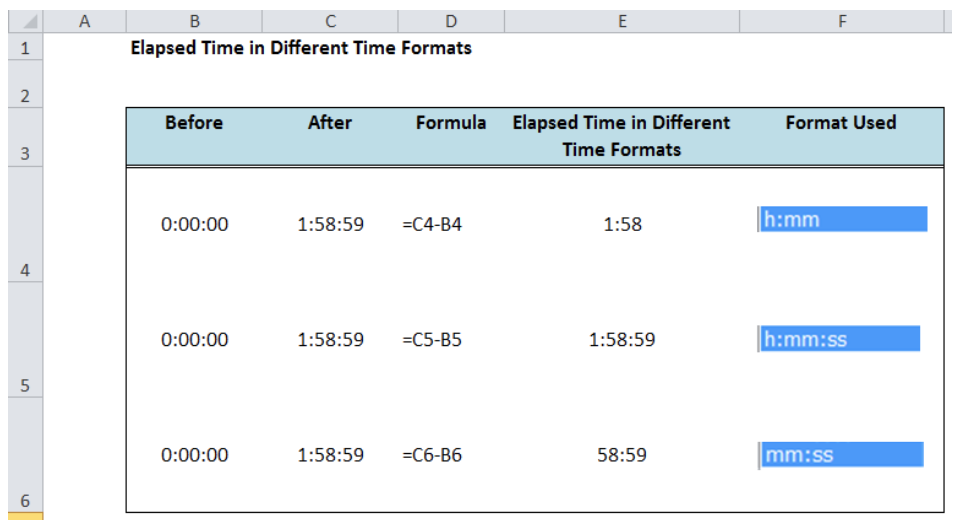Figure 6.  Comparison: Elapsed time in different time formats

Figure 5 above shows how important it is to ensure that the time format we are using is the correct or preferred format.

1. For elapsed time that exceeds 24 hours, the format that we should use is [h]:mm:ss and not h:mm:ss.  The example below shows the difference between the two formats.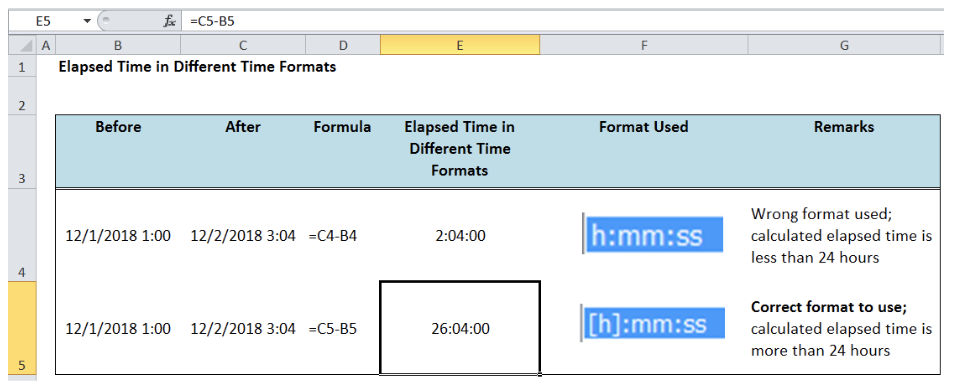Figure 7.  Correct format to use for elapsed time > 24 hours

Most of the time, the problem you will need to solve will be more complex than a simple application of a formula or function. If you want to save hours of research and frustration, try our live Excelchat service! Our Excel Experts are available 24/7 to answer any Excel question you may have. We guarantee a connection within 30 seconds and a customized solution within 20 minutes.

### Did this post not answer your question? Get a solution from connecting with the expert.Another blog reader asked this question today on Excelchat:## Subscribe to Excelchat.coAnother blog reader asked this question today on Excelchat: